Q-Chem 5.0 User’s Manual

# A.3 Eigenvector-Following (EF) Algorithm

The work of Cerjan and Miller , and later Simons and co-workers [897, 898], showed that there was a better step than simply directly following one of the Hessian eigenvectors. A simple modification to the Newton-Raphson step is capable of guiding the search away from the current region towards a stationary point with the required characteristics. This is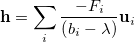(A.6)

in which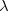can be regarded as a shift parameter on the Hessian eigenvalue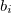. Scaling the Newton-Raphson step in this manner effectively directs the step to lie primarily, but not exclusively (unlike Poppinger’s algorithm ), along one of the local eigenmodes, depending on the value chosen for. References Cerjan:1981,Simons:1983,Banerjee:1985 all utilize the same basic approach of Eq. (A.6) but differ in the means of determining the value of.

The EF algorithm  utilizes the rational function approach presented in Refs. Banerjee:1985, yielding an eigenvalue equation of the form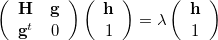(A.7)

from which a suitablecan be obtained. Expanding Eq. (A.7) yields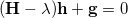(A.8)

and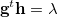(A.9)

In terms of a diagonal Hessian representation, Eq. (A.8) rearranges to Eq. (A.6), and substitution of Eq. (A.6) into the diagonal form of Eq. (A.9) gives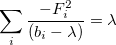(A.10)

which can be used to evaluateiteratively.

The eigenvalues,, of the RFO equation Eq. (A.7) have the following important properties :

• The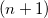values ofbracket theeigenvalues of the Hessian matrix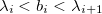.

• At a stationary point, one of the eigenvalues,, of Eq. (A.7) is zero and the othereigenvalues are those of the Hessian at the stationary point.

• For a saddle point of order, the zero eigenvalue separates thenegative and the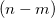positive Hessian eigenvalues.

This last property, the separability of the positive and negative Hessian eigenvalues, enables two shift parameters to be used, one for modes along which the energy is to be maximized and the other for which it is minimized. For a transition state (a first-order saddle point), in terms of the Hessian eigenmodes, we have the two matrix equations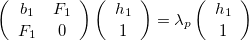(A.11)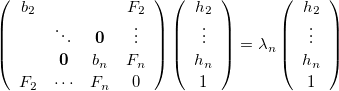(A.12)

where it is assumed that we are maximizing along the lowest Hessian mode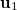. Note that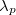is the highest eigenvalue of Eq. (A.11), which is always positive and approaches zero at convergence, and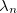is the lowest eigenvalue of Eq. (A.12), which it is always negative and again approaches zero at convergence.

Choosing these values ofgives a step that attempts to maximize along the lowest Hessian mode, while at the same time minimizing along all the other modes. It does this regardless of the Hessian eigenvalue structure (unlike the Newton-Raphson step). The two shift parameters are then used in Eq. (A.6) to give the final step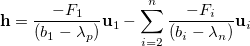(A.13)

If this step is greater than the maximum allowed, it is scaled down. For minimization only one shift parameter,, would be used which would act on all modes.

In Eq. (A.11) and Eq. (A.12) it was assumed that the step would maximize along the lowest Hessian mode,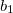, and minimize along all the higher modes. However, it is possible to maximize along modes other than the lowest, and in this way perhaps locate transition states for alternative rearrangements/dissociations from the same initial starting point. For maximization along the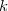th mode (instead of the lowest mode), Eq. (A.11) is replaced by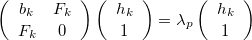(A.14)

and Eq. (A.12) would now exclude theth mode but include the lowest. Since what was originally theth mode is the mode along which the negative eigenvalue is required, then this mode will eventually become the lowest mode at some stage of the optimization. To ensure that the original mode is being followed smoothly from one cycle to the next, the mode that is actually followed is the one with the greatest overlap with the mode followed on the previous cycle. This procedure is known as mode following. For more details and some examples, see Ref. Baker:1986.